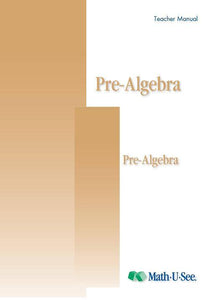or 6 weekly interest-free payments from \$14.83 withwhat's this?Demme Learning

# Math.U.See Pre-Algebra

Regular price \$20.00 \$50.83 Unit price per
Tax included. Shipping calculated at checkout.

## Math.U.See Pre-Algebra

Negative numbers, order of operations, solving for the unknown, and other topics.

Major Concepts and Skills Include:

• Fluently adding, subtracting, multiplying, and dividing positive and negative numbers
• Understanding and simplifying exponents
• Using properties of operations, order of operations, and absolute value
• Expanding understanding of place value
• Identifying the least common multiple and greatest common factor

• Using the Pythagorean theorem to identify triangle attributes
• Transforming temperatures between Celsius and Fahrenheit
• Understanding and computing surface area and volume
• Adding and subtracting time using both 12-hour and military notation